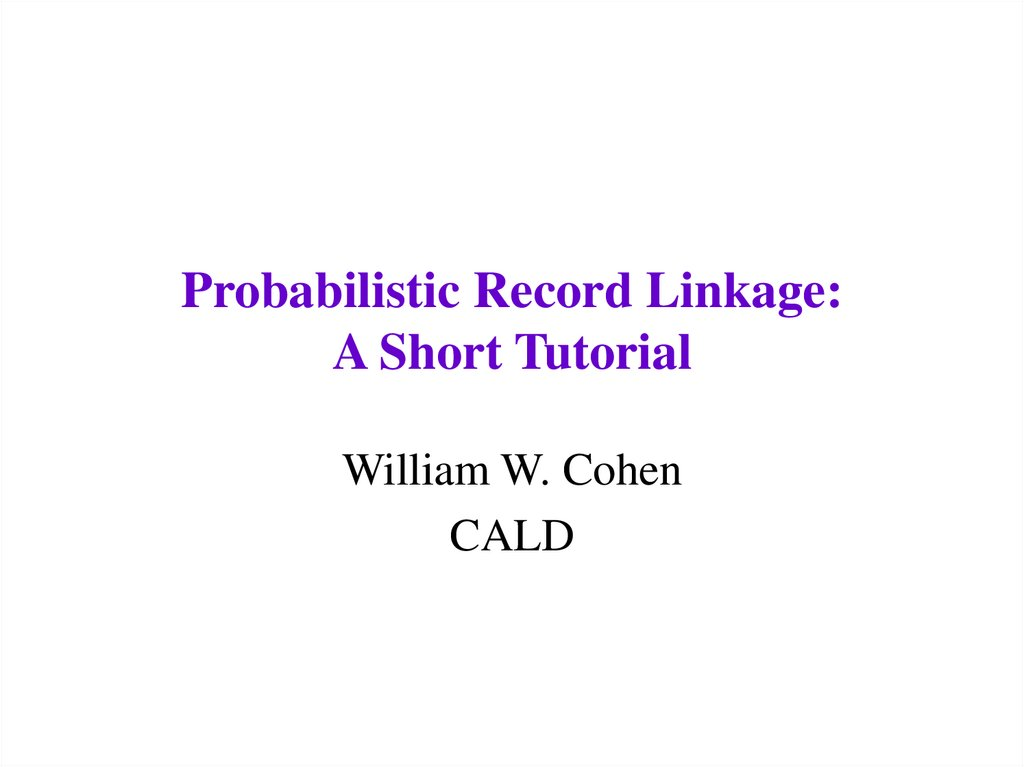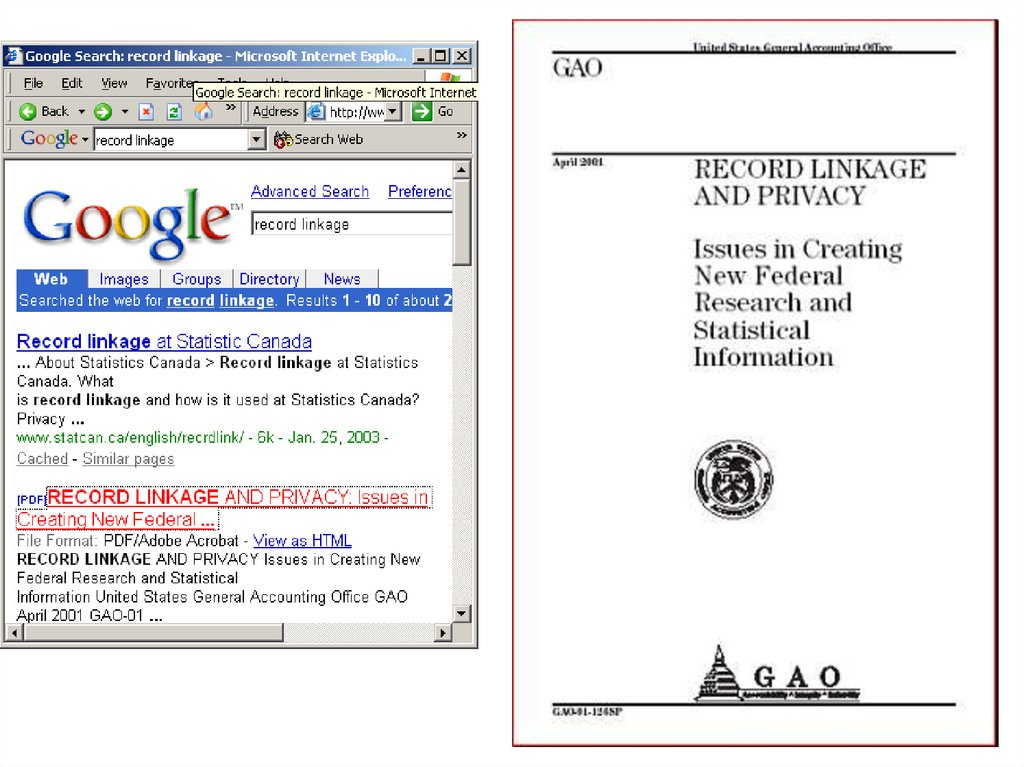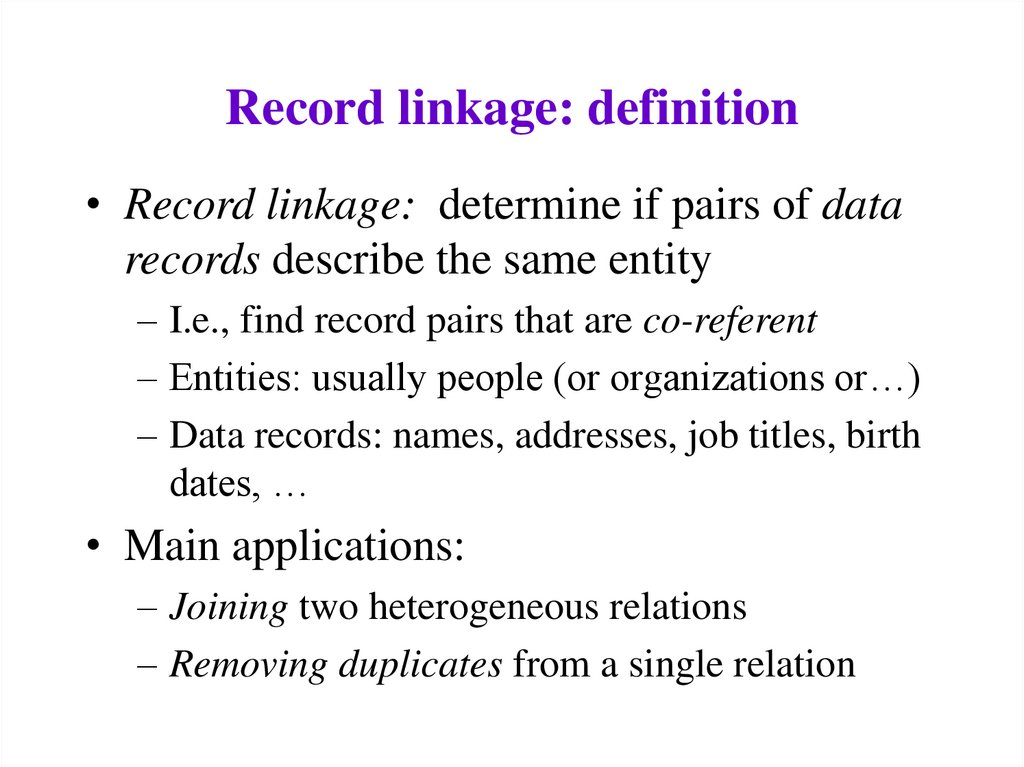# Probabilistic Record Linkage: A Short Tutorial. Matching-1

William W. Cohen
CALD

## 2.

• Record linkage: determine if pairs of data
records describe the same entity
– I.e., find record pairs that are co-referent
– Entities: usually people (or organizations or…)
– Data records: names, addresses, job titles, birth
dates, …
• Main applications:
– Joining two heterogeneous relations
– Removing duplicates from a single relation

• The term “record linkage” is possibly coreferent with:
– For DB people: data matching, merge/purge,
duplicate detection, data cleansing, ETL
– For AI/ML people: reference matching,
database hardening
– In NLP: co-reference/anaphora resolution
– Statistical matching, clustering, language
modeling, …

– This tutorial
– Test equality of normalized version of record
• Normalization loses information
• Very fast when it works!
– Hand-coded rules for an “acceptable match”
• e.g. “same SSNs, or same zipcode, birthdate, and
Soundex code for last name”
• difficult to tune, can be expensive to test

• Toolboxes vs. black boxes:
– To what extent is record linkage an interactive,
exploratory, data-driven process? To what
extent is it done by a hands-off, turn-key,
autonomous system?
• General-purpose vs. domain-specific:
– To what extent is the method specific to a
particular domain? (e.g., Australian mailing

## 7. Record linkage tutorial: outline

• Introduction: definition and terms, etc
• Overview of the Fellegi-Sunter model
• Main issues in Felligi-Sunter model
• Some design decisions
– from original Felligi-Sunter paper
– other possibilities

## 8. Felligini-Sunter: notation

• Two sets to link: A and B
• A x B = {(a,b) : a2A, b2B} = M [ U
– M = matched pairs, U= unmatched pairs
• Record for a2 A is a(a), for b2 B is b(b)
• Comparison vector, written g(a,b), contains
“comparison features” (e.g., “last names are
same”, “birthdates are same year”, …)
– g(a,b)=h g1(a(a),b(b)),…, gK(a(a),b(b))i
• Comparison space G = range of g(a,b)

## 9. Felligini-Sunter: notation

• Three actions on (a,b):
– A1: treat (a,b) as a match
– A2: treat (a,b) as uncertain
– A3: treat (a,b) as a non-match
• A linkage rule is a function
– L: G ! {A1,A2,A3}
• Assume a distribution D over A x B:
– m(g) = PrD( g(a,b) | (a,b)2 M )
– u(g) = PrD( g(a,b) | (a,b)2 U )

## 10. Felligini-Sunter: main result

Suppose we sort all g’s by m(g)/u(g), and pick n< n’ so
n
u (g i )
i 1
N
l m(g i )
i n '
Then the best* linkage rule with Pr(A1|U)= and
Pr(A3|M)=l is:
g1…,gn, gn+1,…,gn’-1,gn’,…,gN
m(g)/u(g) large A
1
A2
A3
m(g)/u(g) small
*Best = minimal Pr(A2)

## 11. Felligini-Sunter: main result

• Intuition: consider changing the action for some gi in the
list, e.g. from A1 to A2.
– To keep constant, swap some gj from A2 to A1.
– …but if u(gj)=u(gi) then m(gj)<m(gi)…
– …so after the swap, P(A2) is increased by m(gi)-m(gj)
mi/ui
mj/uj
g1,…,gi,…,gn,gn+1,…,gj,…,gn’-1,gn’,…,gN
m(g)/u(g) large
A1
A2
A3
m(g)/u(g) small

## 12. Felligini-Sunter: main result

• Allowing ranking rules to be probabilistic means
that one can achieve any Pareto-optimal
combination of ,l with this sort of threshold rule
• Essentially the same result is known as the
probability ranking principle in information
retrieval (Robertson ’77)
– PRP is not always the “right thing” to do: e.g., suppose
the user just wants a few relevant documents
– Similar cases may occur in record linkage: e.g., we just
want to find matches that lead to re-identification

## 13. Main issues in F-S model

• Modeling and training:
– How do we estimate m(g), u(g) ?
• Making decisions with the model:
– How do we set the thresholds and l?
• Feature engineering:
– What should the comparison space G be?
• Distance metrics for text fields
• Normalizing/parsing text fields
• Efficiency issues:
– How do we avoid looking at |A| * |B| pairs?

## 14. Issues for F-S: modeling and training

• How do we estimate m(g), u(g) ?
– Independence assumptions on g=hg1,…,gKi
• Specifically, assume gi, gj are independent given the
class (M or U) - the naïve Bayes assumption
– Don’t assume training data (!)
• Instead look at chance of agreement on “random
pairings”

## 15. Issues for F-S: modeling and training

• Notation for “Method 1”:
– pS(j) = empirical probability estimate for name j
in set S (where S=A, B, AÅB)
– eS = error rate for names in S
• Consider drawing (a,b) from A x B and
measuring gj= “names in a and b are both
name j” and gneq = “names in a and b don’t
match”

## 16. Issues for F-S: modeling and training

• Notation:
– pS(j) = empirical probability estimate for name j in set S
(where S=A, B, AÅB)
– eS = error rate for names in S
• m(gjoe) = Pr( gjoe| M) = pAÅB(joe)(1-eA)(1-eB)
• m(gneq)
1 ( p A B ( j ))(1 e A )(1 eb )
j
1 (1 e A )(1 eb )

## 17. Issues for F-S: modeling and training

• Notation:
– pS(j) = empirical probability estimate for name j in set S
(where S=A, B, AÅB)
– eS = error rate for names in S
• u(gjoe) = Pr( gjoe| U) = pA(joe) pB(joe)(1-eA)(1-eB)
• u(gneq) 1 p A ( j ) pB ( j )(1 e A )(1 eb )
j

## 18. Issues for F-S: modeling and training

• Proposal: assume pA(j)=pB(j)=pAÅ B(j) and estimate from
A[B (since we don’t have AÅB)
• Note: this gives more weight to agreement on rare names
and less weight to common names.

## 19. Issues for F-S: modeling and training

• Aside: log of this weight is same as the inverse
document frequency measure widely used in IR:
m(g joe )
p A B ( joe)
1
log
log
log
joe
u (g )
p A ( joe) pB ( joe)
p( joe)
1
IDF ( joe) log
p( joe)
• Lots of recent/current work on similar IR weighting
schemes that are statistically motivated…

## 20. Issues for F-S: modeling and training

• Alternative approach (Method 2):
– Basic idea is to use estimates for some gi’s to
estimate others
– Broadly similar to E/M training (but less
experimental evidence that it works)
– To estimate m(gh), use counts of
• Agreement of all components gi
• Agreement of gh
• Agreement of all components but gh, i.e. g1,…,gh1,gh+1,gK

## 21. Main issues in F-S: modeling

• Modeling and training: How do we estimate m(g),
u(g) ?
– F-S: Assume independence, and a simple relationship
between pA(j), pB(j) and pAÅ B(j)
• Connections to language modeling/IR approach?
– Or: use training data (of M and U)
• Use active learning to collect labels M and U
– Or: use semi- or un-supervised clustering to find M
and U clusters (Winkler)
– Or: assume a generative model of records a or pairs
(a,b) and find a distance metric based on this
• Do you model the non-matches U ?

## 22. Main issues in F-S model

• Modeling and training:
– How do we estimate m(g), u(g) ?
• Making decisions with the model:
– How do we set the thresholds and l?
• Feature engineering:
– What should the comparison space G be?
• Distance metrics for text fields
• Normalizing/parsing text fields
• Efficiency issues:
– How do we avoid looking at |A| * |B| pairs?

## 23. Main issues in F-S: efficiency

• Efficiency issues: how do we avoid looking at |A|
* |B| pairs?
• Blocking: choose a smaller set of pairs that will
contain all or most matches.
– Simple blocking: compare all pairs that “hash” to the
same value (e.g., same Soundex code for last name,
same birth year)
– Extensions (to increase recall of set of pairs):
• Block on multiple attributes (soundex, zip code) and take union
of all pairs found.
• Windowing: Pick (numerically or lexically) ordered attributes
and sort (e.g., sort on last name). The pick all pairs that appear
“near” each other in the sorted order.

## 24. Main issues in F-S : efficiency

• Efficiency issues: how do we avoid looking at |A|
* |B| pairs?
• Use a sublinear time distance metric like TF-IDF.
– The trick: similarity between sets S and T is
sim ( S , T )
w (t ) w (t )
t S T
S
T
So, to find things like S you only need to look sets T with
overlapping terms, which can be found with an index
mapping S to {terms t in S}
Further trick: to get most similar sets T, need only look at
terms t with large weight wS(t) or wT(t)

## 25. The “canopy” algorithm (NMU, KDD2000)

Input: set S, thresholds BIG, SMALL
Let PAIRS be the empty set.
Let CENTERS = S
While (CENTERS is not empty)
– Pick some a in CENTERS (at random)
– Add to PAIRS all pairs (a,b) such that SIM(a,b)<SMALL
– Remove from CENTERS all points b’ such that
SIM(a,b)<BIG
• Output: the set PAIRS

## 27. Main issues in F-S model

• Making decisions with the model -?
• Feature engineering: What should the
comparison space G be?
– F-S: Up to the user (toolbox approach)
– Or: Generic distance metrics for text fields
• Cohen, IDF based distances
• Elkan/Monge, affine string edit distance
distances

## 28. Main issues in F-S: comparison space

• Feature engineering: What should the
comparison space G be?
– Or: Generic distance metrics for text fields
Bilenko/Mooney
– HMM methods for normalizing text fields
• Example: replacing “St.” with “Street” in addresses,
without screwing up “St. James Ave”
• Seymour, McCallum, Rosenfield
• Christen, Churches, Zhu
• Charniak

## 29. Record linkage tutorial summary

• Introduction: definition and terms, etc
• Overview of Fellegi-Sunter
• Main issues in Felligi-Sunter model
– Modeling, efficiency, decision-making, string
distance metrics and normalization
• Outside the F-S model?
– Form constraints/preferences on match set
– Search for good sets of matches
• Database hardening (Cohen et al KDD2000),
citation matching (Pasula et al NIPS 2002)